# Chairs

In the two dining rooms in the recreational building, there are equally arranged chairs around the tables. A maximum of 78 people can dine in the first dining room and 54 people in the second. How many chairs can be around one table?

Correct result:

x =  6

#### Solution: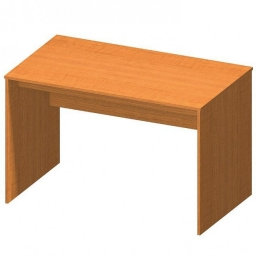We would be pleased if you find an error in the word problem, spelling mistakes, or inaccuracies and send it to us. Thank you!Tips to related online calculators
Do you want to calculate greatest common divisor two or more numbers?

## Next similar math problems:

• Dining tables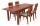In the dining room are tables with 4 chairs, 6 chairs, 8 chairs. How many diners must be at least to be occupy all tables (chairs) and diners are more than 50?
• GroupsIn the 6th class there are 60 girls and 72 boys. We want to divide them into groups so that the number of girls and boys is the same. How many groups can you create? How many girls will be in the group?
• Trees in alleyThere are four trees in the alley between which the distances are 35m, 15m and 95m. Trees must be laid in the spaces so that the distance is the same and the maximum. How many trees will they put in and what will be the distance between them?1st blade 2,5 m, 2nd blade. .1.75 m. How many same long pieces of this two blades can be do the biggest? How long is one piece?
• Around the flowerbed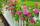Around a rectangular flowerbed with dimensions 5.25 m and 3.5 m are to be planted roses equally spaced so that the roses found in every corner of the flowerbed and consumed them as little as possible. a) At what distance are planted roses? b) How many ros
• Dining roomThe dining room has 11 tables (six and eight seats). In total there are 78 seats in the dining room. How many are six-and eight-seat tables?
• Eight chairsThere are eight chairs by the table and one more child sitting on each one. There are two times more girls than boys. How many girls and how many boys can there be?
• Largest squaresHow many of the largest square sheets did the plumber cut the honeycomb from 16 dm and 96 dm?
• PlumsIn the bowl are plums. How many would be there if we can divide it equally among 8, 10 and 11 children?
• Bicycle wheels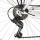Driving wheel of a bicycle has 54 teeth. The driven wheel has 22 teeth. After how many revolutions will meet the same teeth?
• Rectangular flowerbedAround the rectangular flowerbed with dimensions of 5.25 m and 3.50 m, roses should be planted at the same distance from each other so that the roses are located in each corner of the flower bed and are consumed as little as possible. How far do we plant
• PegsFrom two sticks 240 cm and 210 cm long, it is necessary to cut the longest possible pegs for flowers so that no residues remain. How many pegs will it be?
• Common divisorsFind all common divisors of numbers 30 and 45.
• Florist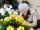Florist has 84 red and 48 white roses. How many same bouquets can he make from them if he must use all roses?
• Square gardensThe gardening colony with dimensions of 180 m and 300 m is to be completely divided into equally large square areas with the largest possible area. Calculate how many such square areas can be obtained and determine the side length of the square.
• Bundle of candies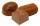In the store has 168 chocolates, caramel candies 224 and 196 hard candies. How many packages we can do and how many of candies will be in each package?
• Dividing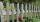Divide the three line segment 13 cm, 26 cm and 19.5 cm long for parts so that the individual parts were equally long and longest. How long will the individual parts and how many it will?